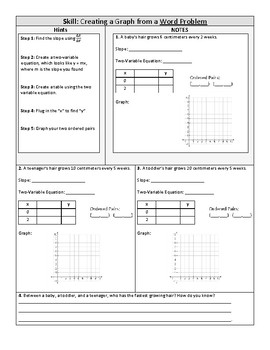# Calculating Slope Notes & Practice (From a table, a graph, a word problem)Subject
Resource Type
File Type

PDF

(509 KB|3 pages)
Standards
Also included in:
1. These Function products (mainly y = mx + b) will last you many days! There are multitude of resources that can be used flexibly to meet the needs of your students. Products include lessons, reference sheets, differentiated stations, scavenger hunts, self-guided worksheets, and culturally-responsive
\$67.25
\$47.08
Save \$20.17
• Product Description
• Standards

For each page, there are hints and a "notes" problem for students to either learn or review finding slope from a table, a graph, and a word problem. I used this as classwork/homework, as well as small group instruction. Enjoy!

Check out my Functions Bundle for more resources!

Interpret the equation 𝘺 = 𝘮𝘹 + 𝘣 as defining a linear function, whose graph is a straight line; give examples of functions that are not linear. For example, the function 𝘈 = 𝑠² giving the area of a square as a function of its side length is not linear because its graph contains the points (1,1), (2,4) and (3,9), which are not on a straight line.
Graph proportional relationships, interpreting the unit rate as the slope of the graph. Compare two different proportional relationships represented in different ways. For example, compare a distance-time graph to a distance-time equation to determine which of two moving objects has greater speed.
Total Pages
3 pages
Not Included
Teaching Duration
40 minutes
Report this Resource to TpT
Reported resources will be reviewed by our team. Report this resource to let us know if this resource violates TpT’s content guidelines.